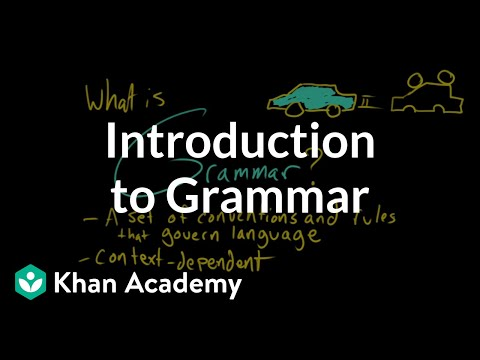Courses

# Test: Egular Grammar

## 15 Questions MCQ Test Compiler Design | Test: Egular Grammar

Description
This mock test of Test: Egular Grammar for Computer Science Engineering (CSE) helps you for every Computer Science Engineering (CSE) entrance exam. This contains 15 Multiple Choice Questions for Computer Science Engineering (CSE) Test: Egular Grammar (mcq) to study with solutions a complete question bank. The solved questions answers in this Test: Egular Grammar quiz give you a good mix of easy questions and tough questions. Computer Science Engineering (CSE) students definitely take this Test: Egular Grammar exercise for a better result in the exam. You can find other Test: Egular Grammar extra questions, long questions & short questions for Computer Science Engineering (CSE) on EduRev as well by searching above.
QUESTION: 1

### How many strings of length less than 4 contains the language described by the regular expression (x+y)*y(a+ab)*?

Solution:

String of length 0 = 1
string of length 1 = 4
string of length 2 = 3
string of length 3 = 3.

QUESTION: 2

Solution:

None.

QUESTION: 3

### A language is regular if and only if

Solution:

All of above machine can accept regular language but all string accepted by machine is regular only for DFA.

QUESTION: 4

Regular grammar is

Solution:

Regular grammar is subset of context free grammar.

QUESTION: 5

Which of the following is not a regular expression?

Solution:

Except b all are regular expression*.

QUESTION: 6

Regular expression are

Solution:

According to Chomsky hierarchy .

QUESTION: 7

Which of the following is true?

Solution:

None.

QUESTION: 8

L and ~L are recursive enumerable then L is

Solution:

If L is recursive enumerable and its complement too if and only if L is recursive.

QUESTION: 9

Regular expressions are closed under

Solution:

According to definition of regular expression.

QUESTION: 10

Consider the production of the grammar S->AA A->aa A->bb Describe the language specified by the production grammar.

Solution:

The production rules give aaaa or aabb or bbaa or bbbb.

QUESTION: 11

Give a production grammar that specified language L = {ai b2i >= 1}

Solution:
QUESTION: 12

Let R1 and R2 be regular sets defined over alphabet ∑ then

Solution:

Union of 2 regular languages is regular.

QUESTION: 13

Which of the following String can be obtained by the language L = {ai b2i / i >=1}

Solution:

Above production rule gives suppose if 3 a’s the corresponding b’s are 6 b’s.

QUESTION: 14

Give a production grammar for the language L = {x/x ∈ (a,b)*, the number of a’s in x is multiple of 3}.

Solution:

The above given condition is satisfied by
S->bS S->B
S->aA s->bA A->aB B->bB
B->aS S->a.

QUESTION: 15

The production Grammar is {S->aSbb, S->abb} is

Solution:

Type 2 grammar satisfies this production grammar.

Track your progress, build streaks, highlight & save important lessons and more!

### Similar Content### Related tests